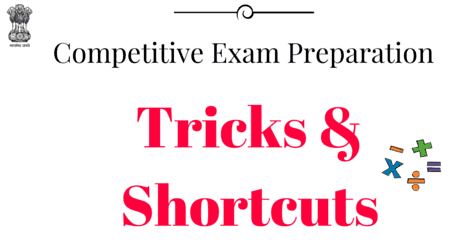# Best Mathematics and Aptitude-Reasoning Shortcuts For All Type Of Competitive Exam like GATE, CAT, Entrance Examinations

The Quantitative Aptitude test measures the numerical ability and accuracy in mathematical calculations. The questions range from purely numeric calculations to problems of arithmetic reasoning, graph and table reading, percentage analysis, categorization and quantitative analysis The quantitative aptitude test measures the numerical ability and accuracy in mathematical calculations. The questions range from purely numeric calculations to problems of arithmetic reasoning, graph and table reading, percentage analysis, categorization and quantitative analysis.Best Mathematics and Aptitude-Reasoning Shortcuts For All Type Of Competitive Exam
It is part of the most competitive exams in India to assess the candidates abilities which plays a key role in selection. It is now a preliminary step in campus recruitment exams across the country for Engineering students. Mastering quant is a must if you want to get through the initial hurdles.

So if you are looking to crack any competitive exams with Quantitative aptitude being part of the syllabus then here are the best shortcut  books you can follow to crack the quantitative Aptitude section.

## Short cut methods in quantitative aptitude-aptitude tricks

1. Aptitude shortcuts pdf

101 Shortcut Book For Quantitative aptitude free Download –This Book will helpful who looking for how to solve quantitative aptitude problem faster

File Size: 400 kb

2) Maths Shortcut tricks for competitive exam

Download below two pdf which include all types of mathematics formulas and shortcut ,maths tricks ,maths shortcut tricks for competitive exam, maths shortcut tricks pdf free download,maths tricks for fast calculation pdf
• 1300 Maths Formulas and Shortcut Book

File Size: 8 mb

• Maths Shortcut Handwritten Notes For Competitive Exams
File Size: 1.6 mb

3)  Logical Reasoning Shortcut Tricks

File Size: 1.2 mb